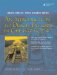# Introduction to Arrays

An array is a sequence of contiguous memory cells, all of the same size. Each cell is called an array element, or entry.

When an array is declared, the size of the array must be made known. This can be done by explicitly or by initialization:

```int a; // explicitly creates uninitialized cells a,
// a,..., a
int b[] = {1, 3, 5, 7}; // implicitly creates and initializes
// b,..., b
```

The array name is an alias for a const typed pointer to the first cell of the array. A pointer declaration such as

```int* ptr;
```

only creates the pointer variable. There is no automatic default initialization of pointer variables. It is an error to attempt to dereference an uninitialized pointer.

Array indices are relative offsets from the base address:

```a[k] is equivalent to *(a + k)
```

The following bit of code demonstrates an interesting aspect of array indices.

```#include
int main(){
int a[] = {10, 11, 12, 13, 14, 15};
int* b = a + 1;
cout << "a = " << a << endl
<< "b = " << b << endl;
}
```

Its output looks like this.

```a = 13
b = 14
```

Notice that b was not declared as an array, but we can use the [] operator anyway.

There is a special syntax for defining a dynamic array consisting of a given number of elements of some type.

```uint n;
ArrayType* pt;
pt = new ArrayType[n];
```

This version of new allocates n contiguous blocks of memory, each of size sizeof(ArrayType) and returns a pointer to the first block. Each element of the newly allocated array is given default initialization. To properly deallocate this array, it is necessary to use the syntax:

```delete[] pt;
```

Using delete without the empty brackets to delete a dynamic array produces undefined results.

### Pointer ArithmeticAn Introduction to Design Patterns in C++ with Qt 4
ISBN: 0131879057
EAN: 2147483647
Year: 2004
Pages: 268